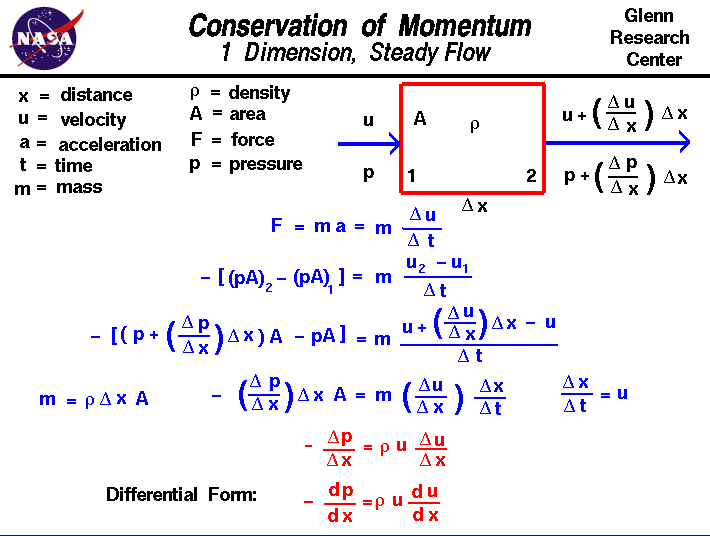+ Text Only Site
+ Non-Flash Version
+ Contact GlennThe conservation of momentum is a fundamental concept of physics along with the conservation of energy and the conservation of mass. Momentum is defined to be the mass of an object multiplied by the velocity of the object. The conservation of momentum states that, within some problem domain, the amount of momentum remains constant; momentum is neither created nor destroyed, but only changed through the action of forces as described by Newton's laws of motion. Dealing with momentum is more difficult than dealing with mass and energy because momentum is a vector quantity having both a magnitude and a direction. Momentum is conserved in all three physical directions at the same time. It is even more difficult when dealing with a gas because forces in one direction can affect the momentum in another direction because of the collisions of many molecules. On this slide, we will present a very, very simplified flow problem where properties only change in one direction. The problem is further simplified by considering a steady flow which does not change with time and by limiting the forces to only those associated with the pressure. Be aware that real flow problems are much more complex than this simple example. Let us consider the flow of a gas through a domain in which flow properties only change in one direction, which we will call "x". The gas enters the domain at station 1 with some velocity u and some pressure p and exits at station 2 with a different value of velocity and pressure. For simplicity, we will assume that the density r remains constant within the domain and that the area A through which the gas flows also remains constant. The location of stations 1 and 2 are separated by a distance called del x. (Delta is the little triangle on the slide and is the Greek letter "d". Mathematicians often use this symbol to denote a change or variation of a quantity. The web print font does not support the Greek letters, so we will just call it "del".) A change with distance is referred to as a gradient to avoid confusion with a change with time which is called a rate. The velocity gradient is indicated by del u / del x; the change in velocity per change in distance. So at station 2, the velocity is given by the velocity at 1 plus the gradient times the distance. u2 = u1 + (del u / del x) * del x A similar expression gives the pressure at the exit: p2 = p1 + (del p / del x) * del x Newton's second law of motion states that force F is equal to the change in momentum with respect to time. For an object with constant mass m this reduces to the mass times acceleration a. An acceleration is a change in velocity with a change in time (del u / del t). Then: F = m * a = m * (del u / del t) The force in this problem comes from the pressure gradient. Since pressure is a force per unit area, the net force on our fluid domain is the pressure times the area at the exit minus the pressure times the area at the entrance. F = - [(p * A)2 - (p * A)1] = m * [(u2 - u1) / del t] The minus sign at the beginning of this expression is used because gases move from a region of high pressure to a region of low pressure; if the pressure increases with x, the velocity will decrease. Substituting for our expressions for velocity and pressure: - [{(p + (del p / del x) * del x} * A) - (p * A)] = m * [(u + (del u / del x) * del x - u) / del t] Simplify: - (del p / del x) * del x * A = m * (del u / del x) * del x / del t Noting that (del x / del t) is the velocity and that the mass is the density r times the volume (area times del x): - (del p / del x) * del x * A = r * del x * A * (del u / del x) * u Simplify: - (del p / del x) = r * u * (del u / del x) The del p / del x and del u / del x represent the pressure and velocity gradients. If we shrink our domain down to differential sizes, these gradients become differentials: - dp/dx = r * u * du/dx This is a one dimensional, steady form of Euler's Equation. It is interesting to note that the pressure drop of a fluid (the term on the left) is proportional to both the value of the velocity and the gradient of the velocity. A solution of this momentum equation gives us the form of the dynamic pressure that appears in Bernoulli's Equation. Activities: Guided ToursBasic Fluid Dynamics Equations:Navigation ..Beginner's Guide Home Page+ Inspector General Hotline + Equal Employment Opportunity Data Posted Pursuant to the No Fear Act + Budgets, Strategic Plans and Accountability Reports + Freedom of Information Act + The President's Management Agenda + NASA Privacy Statement, Disclaimer, and Accessibility CertificationEditor: Nancy Hall NASA Official: Nancy Hall Last Updated: May 13 2021 + Contact Glenn